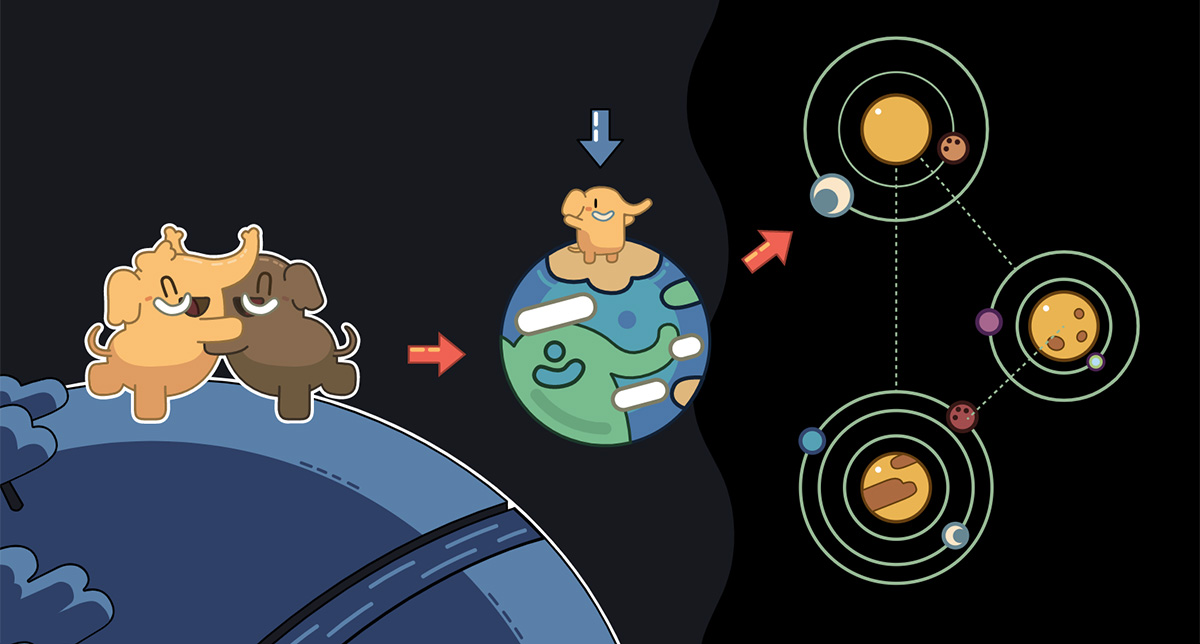Rectangle Regions

I wrote a program in Processing that generates randomized abstracts in the Mondrian style. It uses a framebuffer with integer "pixels" corresponding to pixels in the drawing area, and it takes rectangular regions and increments the numbers in those regions by a random integer. It then computes the modulus base 7 for each pixel and transfers the corresponding color pixel from the framebuffer to the drawing area.

tipsy_tubes.pde

Source code for the Tipsy Tubes series.

Example of one generation: mstdn.social/@Phracker2Art/108

As you can see, I completely winged it on the color gradients, because I'm clueless about how color works in Processing. All I know is that the colors you get roughly correspond to the RRGGBB hexadecimal codes.

Tipsy Tubes

Made this in Processing by repeatedly drawing circles at regular (randomized) offsets and incrementing the color to produce a gradient. The circles line up to produce the illusion of 3D tubes.

Noise Bullseye

Experimenting with semi-random noise in Processing. In this generative art piece I programmed it so that each pixel has a random lightness and the lightness is biased towards a certain value. That value is determined by the sine function, which is plotted in polar coordinates.

You'll notice that there are rings of pure black... This is due to the value of the sine function being zero (I used sin( r/20 ) + 1 so the the area where the sine is zero is stretched out) and the fact that the lightness of each pixel is obtained by multiplying by the sine function. So the lightness of these pixels is a random value multiplied by the sine at that location, which ends up being zero.

I like how the result ended up looking like a 3D styrofoam bullseye.